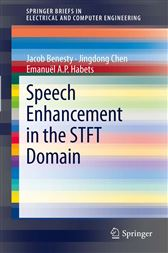Speech Enhancement in the STFT Domain

Series: SpringerBriefs in Electrical and Computer EngineeringCA\$68.48
This work addresses this problem in the short-time Fourier transform (STFT) domain. We divide the general problem into five basic categories depending on the number of microphones being used and whether the interframe or interband correlation is considered. The first category deals with the single-channel problem where STFT coefficients at different frames and frequency bands are assumed to be independent. In this case, the noise reduction filter in each frequency band is basically a real gain. Since a gain does not improve the signal-to-noise ratio (SNR) for any given subband and frame, the noise reduction is basically achieved by liftering the subbands and frames that are less noisy while weighing down on those that are more noisy. The second category also concerns the single-channel problem. The difference is that now the interframe correlation is taken into account and a filter is applied in each subband instead of just a gain.
The advantage of using the interframe correlation is that we can improve not only the long-time fullband SNR, but the frame-wise subband SNR as well. The third and fourth classes discuss the problem of multichannel noise reduction in the STFT domain with and without interframe correlation, respectively. In the last category, we consider the interband correlation in the design of the noise reduction filters. We illustrate the basic principle for the single-channel case as an example, while this concept can be generalized to other scenarios. In all categories, we propose different optimization cost functions from which we derive the optimal filters and we also define the performance measures that help analyzing them.

In The Press

From the reviews:

“This work addresses the problem in the short-time Fourier transform (STFT) domain. The general problem is divided into five basic categories depending on the number of microphones being used and whether the interframe or interband correlation is considered. … This book is mainly a research book for people doing research in electrical and computer engineering.” (Yuehua Wu, Zentralblatt MATH, Vol. 1242, 2012)